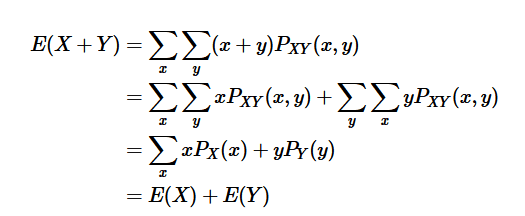# Sum of the Expected Values of Two Discrete Random Variables

• TheBigDig
In summary, the expected value of aX and bY is aE[X]+bE[Y] where X and Y are random variables and a and b are constants.

#### TheBigDig

Homework Statement
Prove that E[aX+bY] = aE[X]+bE[Y]
Relevant Equations
$$E[X] = \sum_{x=0}^{\infty} x p(x)$$
Apologies if this isn't the right forum for this. In my stats homework we have to prove that the expected value of aX and bY is aE[X]+bE[Y] where X and Y are random variables and a and b are constants. I have come across this proof but I'm a little rusty with summations. How is the jump from the second line to the third line made?The probability, ##P_X(x)##, for X taking a fixed value, x, is the sum over all values of Y of ##P_{XY}(x,y)##. So that is substituted in the third line. Likewise, the sum over all values of X of ##P_{XY}(x,y)## is replaced by ##P_Y(y)##.

It's because$$\sum_y x P_{XY}(x,y) = x \sum_y P_{XY}(x,y) = xP_X(x)$$where the second equality follows from the law of total probability.

N.B. I think you're missing a ##\sum## before the ##y P_Y(y)##

TheBigDig said:
How is the jump from the second line to the third line made?

Have you studied joint distributions and their associated "marginal distributions"?

As an example, suppose ##P_{X,Y}## is given by
##P_{X,Y}(1,1) = 0.4##
##P_{X,Y} (1,2) = 0.2##
##P_{X,Y} (2,1) = 0.3##
##P_{X,Y}(2,3) = 0.1##

The associated marginal distribution for ##X## is:
##P_X(1) = 0.6 = P_{X,Y}(1,1) + P_{X,Y}(1,2)##
##P_X(2) = 0.4##

The term ##\sum_x \sum_y x P_{X,Y}(x,y)## denotes:

##( (1) ( P_{X,Y}(1,1) + (1)P_{X,Y}(1,2) ) + ( (2) P_{X,Y}(2,1) + (2)P_{X,Y}(2,2))##
## = (1) P_X(1) + (2)P_X(x)##

In the next line, the term ##\sum_x x P_X(x)## also denotes
## (1) P_X(1) + (2)P_X(2) ##

Stephen Tashi said:
Have you studied joint distributions and their associated "marginal distributions"?

As an example, suppose ##P_{X,Y}## is given by
##P_{X,Y}(1,1) = 0.4##
##P_{X,Y} (1,2) = 0.2##
##P_{X,Y} (2,1) = 0.3##
##P_{X,Y}(2,3) = 0.1##

The associated marginal distribution for ##X## is:
##P_X(1) = 0.6 = P_{X,Y}(1,1) + P_{X,Y}(1,2)##
##P_X(2) = 0.4##

The term ##\sum_x \sum_y x P_{X,Y}(x,y)## denotes:

##( (1) ( P_{X,Y}(1,1) + (1)P_{X,Y}(1,2) ) + ( (2) P_{X,Y}(2,1) + (2)P_{X,Y}(2,2))##
## = (1) P_X(1) + (2)P_X(x)##

In the next line, the term ##\sum_x x P_X(x)## also denotes
## (1) P_X(1) + (2)P_X(2) ##

Okay yes, this definitely seems like something I need to read up on. Our instructor is a little handwavy at the moment saying we'll come across these concepts later but I'm one of those people who needs to understand each element.

etotheipi said:
It's because$$\sum_y x P_{XY}(x,y) = x \sum_y P_{XY}(x,y) = xP_X(x)$$where the second equality follows from the law of total probability.

N.B. I think you're missing a ##\sum## before the ##y P_Y(y)##

Thank you as well. Yes I think it is missing that. I found it online and just copied and pasted the image.